CLAT  >  Test: Average- 1

# Test: Average- 1 - CLAT

Test Description

## 20 Questions MCQ Test Quantitative Techniques for CLAT - Test: Average- 1

Test: Average- 1 for CLAT 2023 is part of Quantitative Techniques for CLAT preparation. The Test: Average- 1 questions and answers have been prepared according to the CLAT exam syllabus.The Test: Average- 1 MCQs are made for CLAT 2023 Exam. Find important definitions, questions, notes, meanings, examples, exercises, MCQs and online tests for Test: Average- 1 below.
Solutions of Test: Average- 1 questions in English are available as part of our Quantitative Techniques for CLAT for CLAT & Test: Average- 1 solutions in Hindi for Quantitative Techniques for CLAT course. Download more important topics, notes, lectures and mock test series for CLAT Exam by signing up for free. Attempt Test: Average- 1 | 20 questions in 30 minutes | Mock test for CLAT preparation | Free important questions MCQ to study Quantitative Techniques for CLAT for CLAT Exam | Download free PDF with solutions
 1 Crore+ students have signed up on EduRev. Have you?
Test: Average- 1 - Question 1

### David obtained 76, 65, 82, 67 and 85 marks (out of 100) in English, Mathematics,Physics, Chemistry and Biology What are his average marks ?

Detailed Solution for Test: Average- 1 - Question 1

Average =   (76 + 65 + 82 + 67 + 85 )/ 5 = 375/5   =  75.

So, the correct option is 'A'

Test: Average- 1 - Question 2

### The average of 5 consecutive even numbers A, B, C, D and E is 52. What is the product of B and E?

Detailed Solution for Test: Average- 1 - Question 2

Let the five consecutive even numbers be x,
x + 2, x + 4, x + 6 and x + 8 respectively.
According to the question,
x + x + 2 + x + 4 + x + 6 + x + 8 = 5 × 52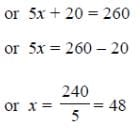B = x + 2 = 48 + 2 = 50 and E = x + 8 = 48 + 8 = 56
B × E = 50 × 56 = 2800

So, the correct option is 'D'

Test: Average- 1 - Question 3

### The average weight of 8 persons increases by 2.5 kg when a new person comes in place of one of them weighing 65 kg. What might be the weight of the new person ?

Detailed Solution for Test: Average- 1 - Question 3

Total weight increased = (8 x 2.5) kg = 20 kg.

Weight of new person = (65 + 20) kg = 85 kg.

So, the correct option is 'C'

Test: Average- 1 - Question 4

Average score of Rahul, Manish & Suresh is 63. Rahul's score is 15 less than Ajay and 10 more than Manish. If Ajay scored 30 marks more than the average score of Rahul, Manish & Suresh, what is the sum of Manish's and Suresh's score?

Detailed Solution for Test: Average- 1 - Question 4

Let score of Rahul, Manish, Suresh, Ajay be r, m, s, a respectively
Average score of Rahul, Manish & Suresh is 63
⇒ Total score of Rahul, Manish & Suresh = 3 x 63 = 189
⇒ r + m + s = 189  —  (i)
Rahul's score is 15 less than Ajay and 10 more than Manish
r = a - 15 —(2)
r = m + 10 — (3)
Ajay scored 30 marks more than the average score of Rahul, Manish & Suresh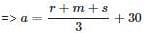⇒ 3a = r + m + s + 90 ----(4)
Using the value of r + m + s from (i) in (4)
3a = 189 + 90
a = 93
Using the value of a in (2)
r = 93 - 15
r = 78
Using the value of r in (i)
78 + m + 3 = 189
m + s = 111
i.e., sum of Manish's and Suresh's score = 111

So, the correct option is 'B'

Test: Average- 1 - Question 5

The average of four consecutive odd numbers is 24. Find the largest number.

Detailed Solution for Test: Average- 1 - Question 5

Let the numbers are x, x+2, x+4, x+6, then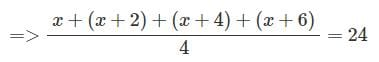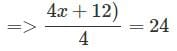=>x+3=24=>x=21
So largest number is 21 + 6 = 27

So, the correct option is 'B'

Test: Average- 1 - Question 6

The average of five positive numbers is 308. The average of first two numbers is 482.5 and the average of last two numbers is 258.5. What is the third number.

Detailed Solution for Test: Average- 1 - Question 6

Let the numbers be A1, A2 , A3 , A4 , A5

► Average of 5 numbers = (A1 + A2 + A3 + A4 + A5) / 5 = 308

► Now A1 + A2 + A3 + A4 + A5 = 308 x 5 = 1540

► Also,A1 + A2 = 482.5 x 2 = 965

and A4 + A5 = 258.5 x 2 = 517

► A3 = 1540 − 965 − 517 = 58

So, the correct option is 'C'

Test: Average- 1 - Question 7

Average age of 54 girls in a class was found to be 10 years. It was later on realised that the actual age of one of the girls in the class was 8 years but it was wrongly taken as 18. The actual average age of the girls in the class is

Detailed Solution for Test: Average- 1 - Question 7

Summation of ages of 54 girls= 54*10= 540 since 18 is mistakely taken in place of 8 so total summation of ages of girls after correction= 540-18+8= 530 Now the actual average age of the girls = 530/54 =9.81

So,the correct option is 'B'

Test: Average- 1 - Question 8

The sum of seven consecutive numbers is 175. What is the sum of the first and the last number

Detailed Solution for Test: Average- 1 - Question 8

Sum of seven consecutive numbers x+(x+1)+(x+2)+(x+3)+(x+4)+(x+5)+(x+6)=175 7x+21=175
7x=154
x=22
First number will be 22 and consecutive series is 22, 23, 24, 25, 26, 27, 28

Sum of first and last number =22+28=50

So, the correct option is 'D'

Test: Average- 1 - Question 9

The average marks of nine students in a group is 63. Three of them scored 78, 69 and 48 marks. What are the average marks of remaining six students?

Detailed Solution for Test: Average- 1 - Question 9

The total marks of nine students = 9 × 63 = 567

Sum of the marks of three students = 78 + 69 + 48 = 195

Therefore, the sum of marks of the remaining six students= 567 – 195 = 372

Average marks of remaining six students = 372/6 = 62.

So, the correct option is 'E'

Test: Average- 1 - Question 10

The average age of 20 boys in a class is 12 years. 5 new boys are admitted to the class whose average age is 7 years. The average age of all the boys in the class becomes

Detailed Solution for Test: Average- 1 - Question 10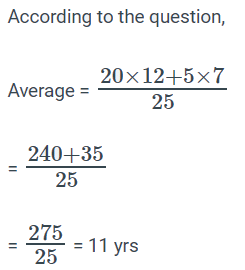So, the correct option is 'A'

Test: Average- 1 - Question 11

The average age of 24 boys and their teacher is 15 years. When the teacher’s age is excluded, the average age decreases by one year. The age of the teacher is

Detailed Solution for Test: Average- 1 - Question 11

Age of the teacher = (25×15−24×14)years
(375−336) years= 39years

So, the correct option is 'C'

Test: Average- 1 - Question 12

The average of five positive numbers is 213. The average of the first two numbers is 233.5 and the average of last two numbers is 271. What is the third number?

Detailed Solution for Test: Average- 1 - Question 12

► The sum of the five numbers = 5 * 213 =1065

► The sum of the first two numbers = 2 * 233.5 = 467

► The sum of  the last two numbers = 542

► Then the sum of the four numbers = 467 + 542 =1009

► So, the third number will be = 1065 – 1009 = 56

So, the correct option is 'B'

Test: Average- 1 - Question 13

The average weight of 8 men is increased by 1.5 kg when one of them whose weight is 65 kg is replaced by a new man. The weight of the new man is

Detailed Solution for Test: Average- 1 - Question 13

Total weight increased = (8 * 1.5) kg = 12 kg.
Weight of new man = 65 + 12 = 77 kg

So, the correct option is 'D'

Test: Average- 1 - Question 14

The average weight of 21 boys was recorded as 64 kg. If the weight of the teacher was added, the average increased by one kg. What was the teacher's weight?

Detailed Solution for Test: Average- 1 - Question 14

Here, n = 21, a = 64, b = 65
Weight of teacher = n (b - a) + b
= 21 (65 - 64) + 65 = 21 + 65 = 86 kg

So, the correct option is 'C'

Test: Average- 1 - Question 15

The average height of 16 students in a class is 142 cm. If the height of the teacher is added the average increases by 1 cm. What is the height of the teacher in cm?

Detailed Solution for Test: Average- 1 - Question 15

Height of the teacher = (17 * 143) − (16 * 142) = 2431 - 2272 = 159 cm

So, the correct option is 'A'

Test: Average- 1 - Question 16

The average weight of 15 girls was recorded as 54 kg. If the weight of the teacher was added, the average increased by two kg. What was the teacher's weight.

Detailed Solution for Test: Average- 1 - Question 16

Given that average is 54 kg for 15 girls.

(Sum of weight of 15 girls) / 15 = 54

Sum of weight of 15 girls = 15 x 54 = 810

Now weight of teacher is also added so the average becomes 54 + 1 kg = 55 kg

(Sum of weight of 15 girls + Weight of teacher ) / (15 girls + 1 teacher)  = New average = 55 (Given)

(810 + Weight of teacher ) / (16) = 55 + 1

810 + Weight of teacher = 16 (56)

Weight of teacher = 896 - 810 = 86 kg

So, the correct option is 'D'

Test: Average- 1 - Question 17

Find the average of first 10 even numbers

Detailed Solution for Test: Average- 1 - Question 17

2+4+6+8+10+12+14+16+18+20/10 = 110/10
=11

So, the correct option is 'A'

Test: Average- 1 - Question 18

Find the average of first 15 odd numbers

Detailed Solution for Test: Average- 1 - Question 18

► The first 15 odd numbers are 1,3,5,7,9,11,13,15,17,19,21,23,25,27,29

► The sum of first 15 odd numbers are 225

► Therefore mean is equal to 225/15=15

► Median =Middle value=15

So, the correct option is 'D'

Test: Average- 1 - Question 19

What will be the average of even numbers between 11 to 63

Detailed Solution for Test: Average- 1 - Question 19

Even numbers between 11 to 63
= 12,14,16,18,20,..........,62.
Clearly, this is a series of consecutive even numbers.

According to the formula,
Average of consecutive even numbers = (First number + Last number)/2 = (12 + 62)/2  = 74/2

So, the correct option is 'D'

Test: Average- 1 - Question 20

The average of 7 consecutive numbers is 20. The largest of these numbers is :

Detailed Solution for Test: Average- 1 - Question 20

Let the numbers be x, x + 1, x + 2,  x + 3, x + 4, x + 5 and x + 6
Then [x + (x + 1) + (x + 2) + (x + 3) + (x + 4) + (x + 5) + (x + 6)] / 7 = 20.
⇒ 7x + 21 = 140
⇒ 7x = 119
⇒ x =17.

Latest number = x + 6 = 23.

So, the correct  option is 'C'

## Quantitative Techniques for CLAT

46 videos|69 docs|95 tests
Information about Test: Average- 1 Page
In this test you can find the Exam questions for Test: Average- 1 solved & explained in the simplest way possible. Besides giving Questions and answers for Test: Average- 1, EduRev gives you an ample number of Online tests for practice

## Quantitative Techniques for CLAT

46 videos|69 docs|95 tests